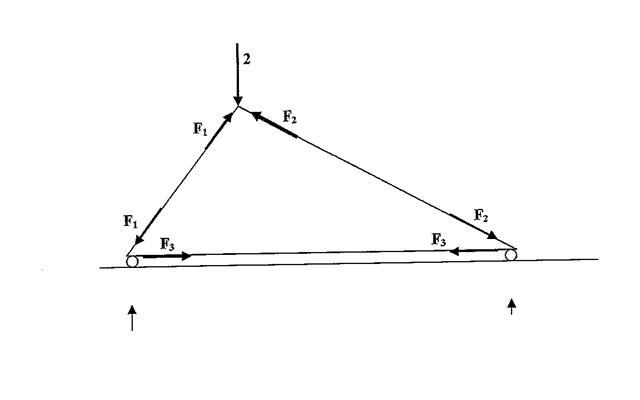# Simultaneous equations?

• Stacyg

#### Stacyg

A weight of 2 T is supported by a three bar truss. We want to find the forces in the three bars.

(This truss is a 3-4-5 triangle so that sin and cos are either 0.6 or 0.8. See below

Resolving vertically at the top we find 0.8F1 + 0.6 F2 = 2 This is Equation 1

Resolving horizontally at the top we find 0.6F1 = 0.8F2 This is equation 2

Resolving horizontally at the bottom left we find 0.6F1 = F3

a) Write each of the equations in th eform p.F1 + r.F2 + r.F3 = k

b) Solve these questions simultaneously.

I have no idea how to do this.
I'll try and put up a picture of the question as soon as possible.
Thanks.

Solve the last equation for F1 in terms of F3 and substitute it into the other equations. Now you have two equations in two unknowns. Solve one of them for F2 in terms of F3 and substitute that into the last equation. Now you have one equation in one unknown. Et voila.

Dick speaks the truth. It is quite common to encounter a system like this that can be solved by 'back-substitution'. Since you've probably not learned about them yet, matrices are how you would normally handle a system of equations such as this one.

DiagramHere is the diagram.
I really don't understand how to solve the equations to find f1, f2 and f3.
Also how would i write the equations in the form p.F1 +q.F2+r.F3=k
Where do the p, q, r, and k that are constants come from ?

Thanks

View attachment 14020

Here is the diagram.
I really don't understand how to solve the equations to find f1, f2 and f3.
Also how would i write the equations in the form p.F1 +q.F2+r.F3=k
Where do the p, q, r, and k that are constants come from ?

Thanks

Your first equation was 0.8F1 + 0.6 F2 = 2. That's p=0.8, q=0.6, r=0 and k=2. Didn't you read my explanation of how to solve them??